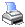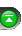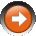﻿ Plots
 NinjaScript > Language Reference > Indicator > PlotsDefinition
A collection holding all of the Plot objects that define the visualization characteristics of the indicator.

Property Value

A collection of Plot objects.

Syntax

 Plots[int index]

Examples

 // Initialize method of a custom indicator protected override void Initialize() {     // Lines are added to the Lines collection in order     Add(new Plot(Color.Orange, "Plot1")); // Stored in Plots     Add(new Plot(Color.Blue, "Plot2"));   // Stored in Plots }   // Dynamically change the primary plot's color based on the indicator value protected override void OnBarUpdate() {     if (Value > 70)          Plots.Pen = new Pen(Color.Blue);     else          Plots.Pen = new Pen(Color.Red); }